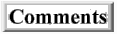Is There a Holographic Principle Here?

Home     TOC

Not just anything can happen in the world. There is at least one constraint. Any event that happens must be consistent with everything else in reality. So the universe contained in the whole volume of space limits what can happen at any particular place. There seems to be some intuition that what happens here on earth is governed by forces far beyond our planet such as astrology, aliens, and gods. All of which speak of forces in the heavens that govern the affairs of men. In modern terms, all these scenarios may be primitive ways of describing a holographic principle, in which the events in our local three dimensional space correlate to what happens in a distance two dimensional sphere surrounding us. The questions is whether there is some way to derive a holographic principle in terms of the framework developed here.

I went the route of putting the Dirac measure in terms of propositions (not sets) labeled with individual coordinates . So I was able to put the Dirac measure in terms of the Kronecker delta and then the Dirac delta function. Expressing the Dirac delta in terms of the complex, gaussian exponential (which is commonly done), I got Feynman’s Path Integral.

However, now it occurs to me to go back to using the Dirac measure in terms of sets and subsets. Then in a coordinate system, sets are expressed as regions of space with a boundary. You can see where this is going. Can I get the same logic of implications expressed in terms of the Dirac measure put in terms of points to also be expressed in terms of the Dirac measure put in terms of sets? In terms of points, I got the dynamics of how a point particle propagates. So can I get the dynamics of how a surface propagates if I express the logic in terms of regions? But what math would I use?

This is a work in progress; I'll have more to say about it soon.